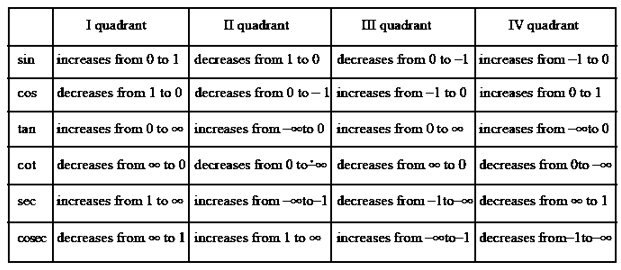# Domain, Graph and Range of Trigonometric Functions

## What is Domain and Range of Trigonometric Functions?

The input and output values of trigonometric functions are the domain and range of trigonometric functions, respectively. We know that there are six trigonometric functions namely

1. $\sin\theta$
2. $\cos\theta$
3. $\tan\theta$
4. $\cot\theta$
5. $\sec\theta$
6. $\csc\theta$

The angle and the resultant value, respectively, define the domain and range of trigonometric functions. The trigonometric functions' domain is angles in degrees or radians, and their range is a real number.

The domain of trigonometric functions specifies the values of angles where the trigonometric functions are defined, whereas the range of trigonometric functions denotes the resultant value of the trigonometric function corresponding to a certain angle in the domain.

## Domain and Range of Sine Function

• $y=f(x)= Sin(x)$
• Domain of Sine Function : It is defined for all real values of $x$ i.e., $(-\infty , \infty)$
• Range of Sine Function: $-1 \leq y \leq 1$
• Period of Sine Function: $2\pi$
• It is a odd function

### Graph of sine function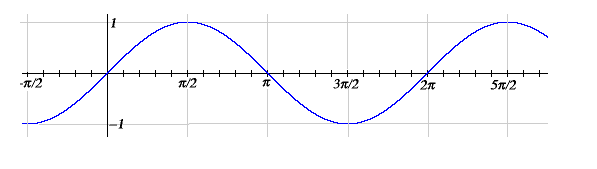More Graphs on sine function for $y=f(x)=2 \sin (x)$ and $y=f(x)=3 \sin (x)$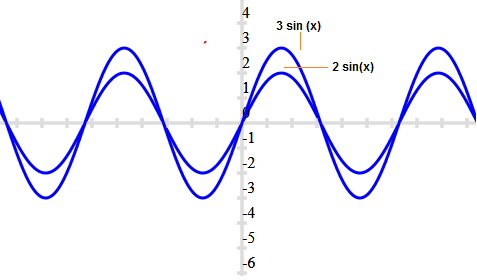We can see that range get increased in the similar way
for $y=f(x)=\sin (2x)$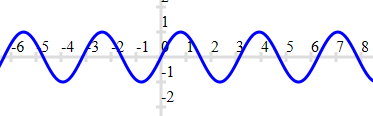We can see that range remains but graph get shrink

## Domain and Range of Cosine Function

• $y=f(x)= cos(x)$
• Domain of Cosine Function: It is defined for all real values of $x$
• Range of Cosine Function: $-1 \leq y \leq 1$
• Period of Cosine Function: $2\pi$
• It is even function

### Graph of cos(x) function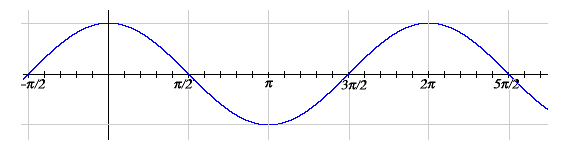More Graphs on contains function for $y=f(x)=2 cos (x)$ and $y=f(x)=3 cos (x)$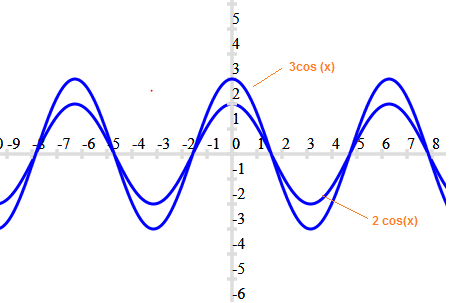We can see that range get increased in the similar way
for $y=f(x)=cos (2x)$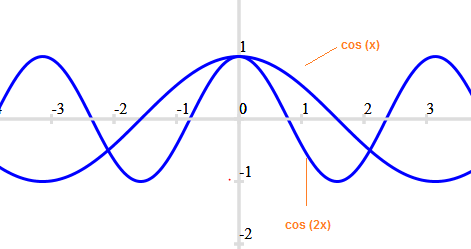We can see that range remains but graph get shrink.

## Domain and Range of Tangent Function

• $y=f(x)=tan(x)$
• Domain of Tangent Function: It is defined for all real values of x except x ≠(2n + 1)(π/2) where n is any integer.
• Range of Tangent Function: All the real numbers
• Period of Tangent Function: π
• It is a odd function
• ### Graph of tan(x) function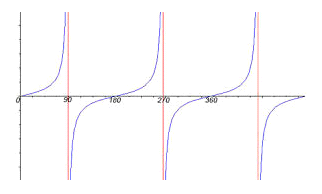## Domain and range of Cotangent Function

• $y=f(x)=cot(x)$
• Domain of Cotangent Function: It is defined for all real values of $x$ except $x \neq n\pi$, where $n$ is any integer
• Range of Cotangent Function: All the real numbers
• Period of Cotangent Function: π
• It is a odd function

### Graph of cot(x) function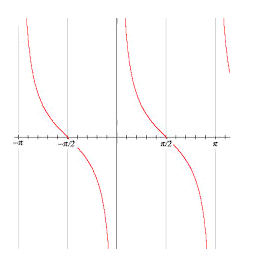## Domain and range of Secant Function

• y=f(x)=sec(x)
• Domain of Secant Function: It is defined for all real values of x except x ≠(2n + 1)(π/2) where n is any integer
• Range of Secant Function: (-∞,-1] ∪ [1,∞)
• Period of Secant Function:
• It is even function

### Graph of sec(x) function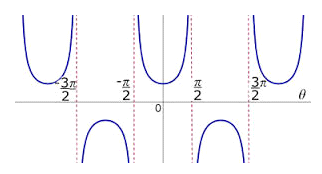## Domain and range of Cosecant Function

• $y=f(x)=cosec(x)$
• Domain of Cosecant Function: It is defined for all real values of x except x ≠nπ, where n is any integer
• Range of Cosecant Function: (-∞,-1] ∪ [1,∞)
• Period of Cosecant Function:
• It is odd function

### Graph of cosec(x) function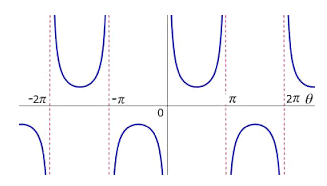## Domain and Range of Trigonometric Functions Table

We can write the range for the trigonometric functions in below summary table